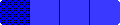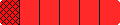Home

Grades 1-2 Fraction Bars Teacher's Guide

 Sample Lessons Grades 1-2 The 1-2 Grades Fraction Bars Guide contains lessons for introducing the following eight topics: Basic Concepts; Equality; Fractions for Regions; Story Problems; Inequality; Fractions for Collections; Number Lines; and Measurement. The first four topics are for grade 1 and all eight topics are for grade 2.Guide with two-page facing lessons Each lesson is on two facing pages and opens with the purpose of the lesson and the materials required.The main component of each lesson is TEACHER MODELING/STUDENT COMMUNICATION (instruction and student activities) and is followed by INDEPENDENT PRACTICE and ASSESSMENT (activity sheets and in some cases interactive online games). There are two story-problem lessons one for the first grade and one for the second grade. Under separate cover is a Grades 1-2 Teacher Resource Package that includes two activity sheets for each lesson and a set of answers for each grade level. The top four links in the following table provide samples of the first pages of First Grade two-page lessons and the bottom four links provide samples of the first pages of Second Grade two-page lessons.

### Measurement

HomeHome